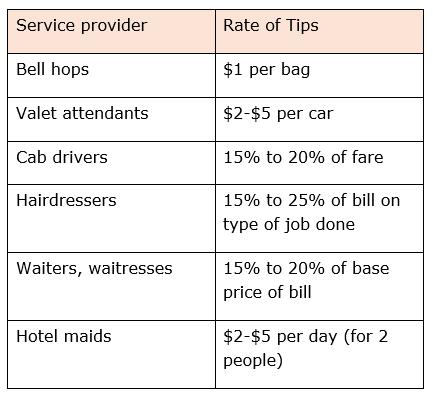# Estimating a tip without a calculator

#### Complete Python Prime Pack

9 Courses     2 eBooks

#### Artificial Intelligence & Machine Learning Prime Pack

6 Courses     1 eBooks

#### Java Prime Pack

9 Courses     2 eBooks

A tip is not a hint or a clue about something at least in real world consumer math. It is a percentage given or paid cash in addition to the base price for a service provided. It is also called as a “gratuity.” There are many professions where giving the service provider a tip is important. In fact, the bulk of their income is dependent upon tips. If no one tipped them their employers would have to pay them more. This, in turns, means the employer would have to charge the public more to make up for the higher wages. So, some professions pay their employees a low wage and those employees then depend upon tips for most of their income. Generally, professions that provide a personalized service use the practice of giving tips.

Tips are given to cab drivers, bell hops, hair dressers, movers, waiters/waitresses, hotel maids, valet attendants, etc.

## Calculating Amount of Tip

Some typical rates of tips given to various service providers are as follows. These may vary from case to case.To estimate a tip without a calculator, we use some mental math methods as shown in solved examples.

To estimate a tip without a calculator, we use some mental math methods as shown in solved examples.

### Solution

Step 1:

Bill amount = $88; For 10% of$88.00, we move decimal one place to left and get $8.80; Step 2: 5% of$88 = $\frac{\$8.80}{2} = \$4.40$

Step 3:

15% of $88 = 10% of$88 + 5% of $88 =$8.80 + $4.40 =$13.20

So, the tip amount = $13.20 Maggie went to a beauty salon to get her hair cut and styled. Her bill came to$115.00. How much did Maggie tip her hairdresser, if she gave 20% tip?

### Solution

Step 1:

Bill amount = $115 Tip = 20% of$115.00

Step 2:

First to get 10% of $115.00, we move the decimal one place to the left to get$11.50

Step 3:

To get 20% we double this amount as

20 = 2 × 10

So, 20% of $115 = 2 ×$11.50 = $23.00 So, the tip amount =$23.00

Thomas pays a parking bill of $48. He decides to leave a 5% tip for the parking guard. How much will the total bill be? ### Solution Step 1: Parking bill amount =$48.00

To get 10% of $48.00%, we shift decimal one place to left and get$4.80

Step 2:

5% is half of 10%.

So, 5% of $48.00 =$\frac{\$4.80}{2} = \$2.40$Step 3: Total bill = bill amount + tip =$48.00 + $2.40 =$50.40